# 时间序列算法理论及python实现（1-算法理论部分）

## 1 时间序列算法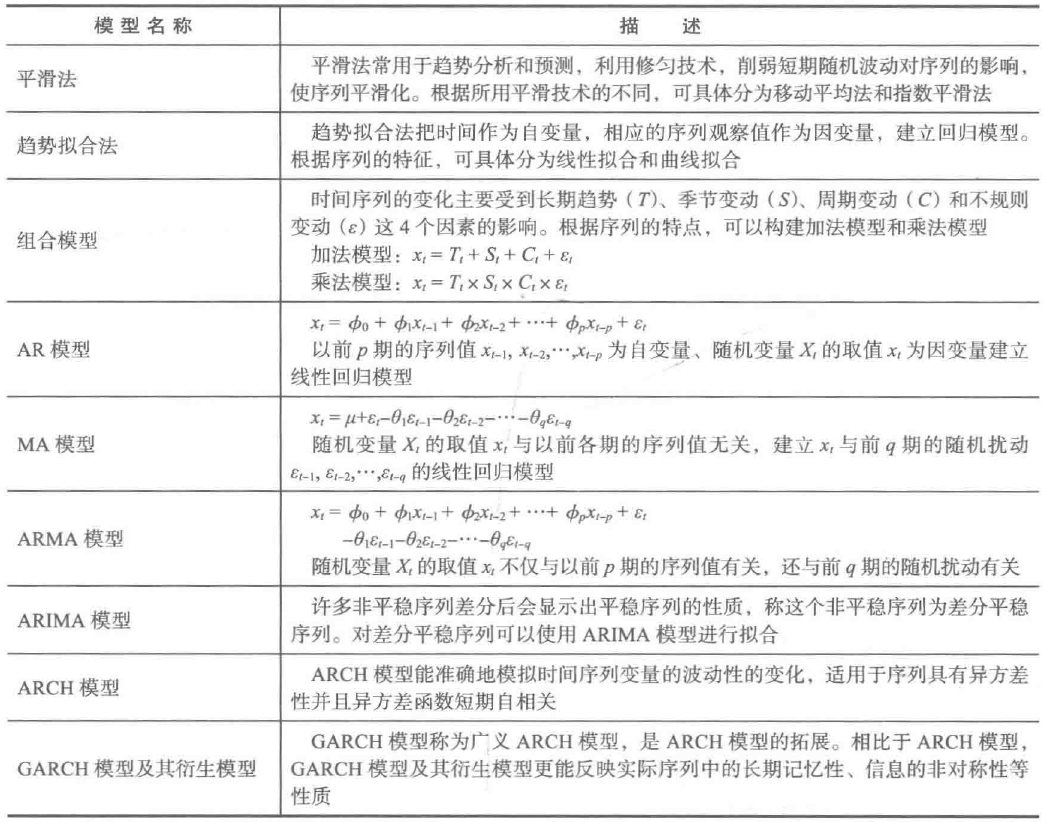(1) 时序图检验

(2) 自相关图检验

(3) 单位根检验

## 3 平稳时间序列分析

ARMA模型的全称是自回归移动平均模型，它是目前最常用的拟合平稳序列的模型。它又可以细分为AR模型、MA模型和ARMA模型三大类。都可以看作是多元线性回归模型。

### 3.1 AR模型

$${x_t} = \mu + \sum\limits_{i = 1}^p {{\phi _i}{x_{t - i}}} + {\varepsilon _t} = {\phi _0} + {\phi _1}{x_{t - 1}} + {\phi _2}{x_{t - 2}} + \cdots + {\phi _p}{x_{t - p}} + {\varepsilon _t}$$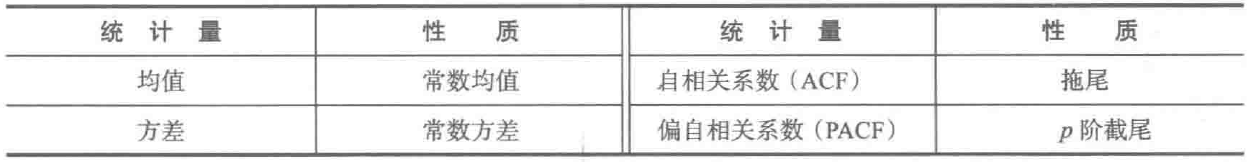#### 3.1.1 均值

$$E({x_t}) = E({\phi _0} + {\phi _1}{x_{t - 1}} + {\phi _2}{x_{t - 2}} + \cdots + {\phi _p}{x_{t - p}} + {\varepsilon _t})$$

### 3.2 MA模型

$${x_t} = \mu + {\varepsilon _t} + \sum\limits_{i = 1}^q {{\theta _i}{\varepsilon _{t - i}}} = \mu + {\varepsilon _t} + {\theta _1}{\varepsilon _{t - 1}} + {\theta _2}{\varepsilon _{t - 2}} + \cdots + {\theta _q}{\varepsilon _{t - q}}$$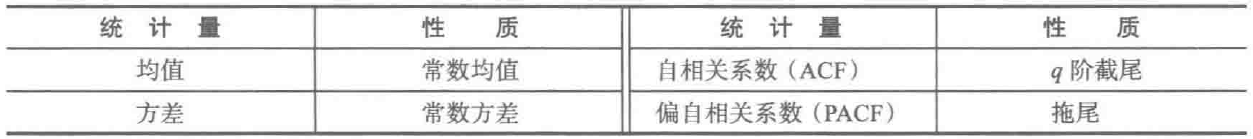### 3.3 ARMA模型

$${x_t} = \mu + \sum\limits_{i = 1}^p {{\phi _i}{x_{t - i}}} + {\varepsilon _t} + \sum\limits_i^q {{\theta _i}{\varepsilon _{t - i}}}$$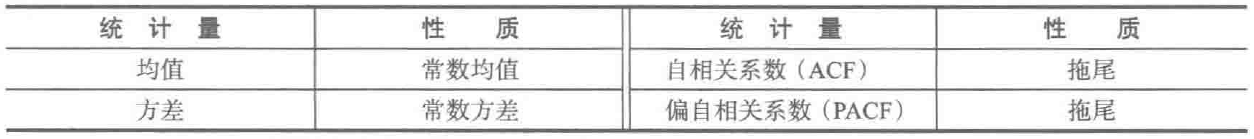### 3.4 相关评判方法介绍

3.4.1 自相关系数（ACF）

#### 3.4.2 偏自相关系数（PACF）

${X_t}$与${X_{t - k}}$之间单纯的相关关系，因为${X_t}$同时还会收到中间$k - 1$个随机变量${X_{t - 1}},{X_{t - 2}}, \cdots ,{X_{t - k}}$的影响，所以自相关系数${\rho _k}$里实际上掺杂了其他变量对${X_t}$与${X_{t - k}}$的相关影响，为了单纯地测度${X_{t - k}}$对${X_t}$的影响，引进偏自相关系数的概念。

#### 3.4.3 截尾和拖尾

（1）p阶自回归模型$AR(p)$

AR(p)模型的偏自相关函数PACF在p阶之后应为零，称其具有截尾性；

AR(p)模型的自相关函数ACF不能在某一步之后为零（截尾），而是按指数衰减（或成正弦波形式)，称其具有拖尾性。

（2）q阶移动平均模型$MA(q)$

MA(q)模型的自相关函数ACF在q阶之后应为零，称其具有截尾性；

MA(q)模型的偏自相关函数PACF不能在某一步之后为零（截尾），而是按指数衰减（或成正弦波形式)，称其具有拖尾性。

#### 3.4.4 截尾和拖尾判断

（1）如果样本自相关系数（或偏自相关系数）在最初的d阶明显大于2倍标准差范围，而后几乎95%的样本自相关（偏自相关）系数都落在2倍标准差范围以内，而且由非零自相关（偏自相关）系数衰减为小值波动的过程非常突然，这时，通常视为自相关（偏自相关）系数截尾。

（2）如果有超过5%的样本相关系数落在2倍标准差范围以外，或者是由显著非零的相关函数衰减为小值波动的过程比较缓慢或者非常连续，这时，通常视为相关系数不截尾。

### 3.4 平稳时间序列建模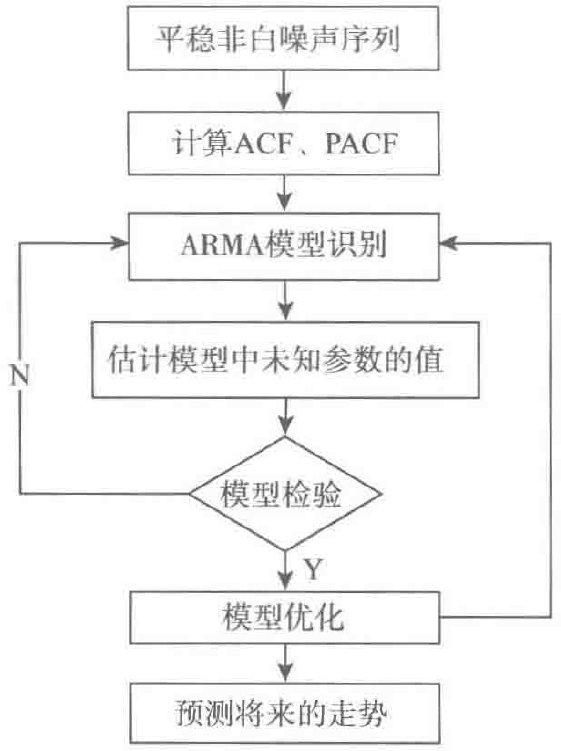#### 3.4.2 ARMA(p,d,q)模型识别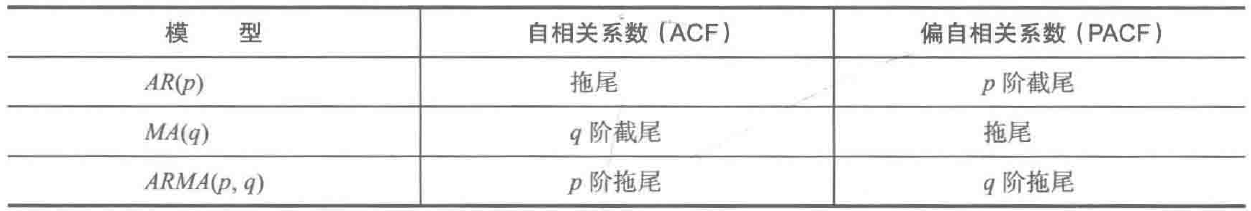## 4 非平稳时间序列分析

### 4.2 ARIMA模型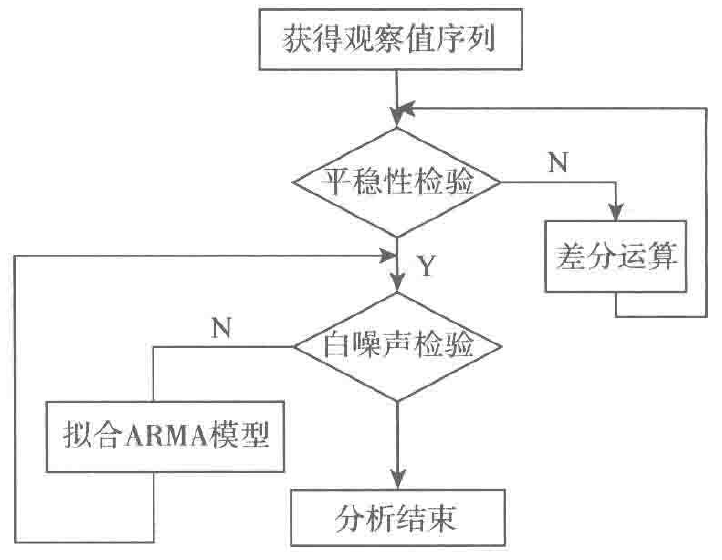#### 4.2.2 ARIMA模型识别#### 4.2.3 使用AIC与BIC模型选择：选择更简单的模型

（1）AIC：赤池信息准则（Akaike Information Criterion，$AIC$）

$$AIC=2k-2ln(L)$$

（2）BIC：贝叶斯信息准则（Bayesian Information Criterion，$BIC$）

$$BIC=kln(n)-2ln(L)$$

#### 4.2.4 模型检验

（1）残差检验分析

• 残差图肉眼简单查看；
• ARIMA模型的残差是否是平均值为0且方差为常数的正态分布；
• QQ图：线性即正态分布；
• Ljung-Box检验：独立性

（2）过度拟合和参数冗余

• 在过度拟合时，不要同时增加AR和MA部分的阶数
• 例如：如果拟合了MA(1)模型后，残差在2阶滞后处仍存在明显的相关性，那么应该尝试MA(2)，而不是ARMA(1,1)模型。

## 5 Python实现ARIMA模型

由于写在同一篇博客篇幅太长，不利用阅读，这部分内容请移步我的另一篇博文：时间序列算法理论及python实现（2-python实现） - 知-青 - 博客园

## 6 Python主要时序模式算法

同理，由于写在同一篇博客篇幅太长，不利用阅读，这部分内容请移步我的另一篇博文：

## 7 文献

python时间序列分析 - 大熊猫淘沙 - 博客园

ARIMA模型的拖尾截尾问题 - CSDN博客

Complete guide to create a Time Series Forecast (with Codes in Python)1、本人博客纯属技术积累和分享，欢迎大家评论和交流以求共同进步。

2、在无明确说明下，博客可以转载以供个人学习和交流，但是要附上出处。

3、如果原创博客使用涉及商业/公司行为请邮件(1547364995@qq.com)告知，一般情况均会及时回复同意。

4、如果个人博客中涉及他人文章我会尽力注明出处，但受限于能力并不能保证所有引用之处均能够注明出处，如有冒犯，请您及时邮件告知以便修改，并于此提前向您道歉。

5、转载过程中如有涉及他人作品请您与作者联系。

6、所有文章（不限于原创）仅为个人见解，个人只能尽量保证正确，如有错误您需要自负责任，并请您留下评论提出错误之处以便及时更正，惠泽他人，谢谢

posted @ 2018-07-09 16:14  知-青  阅读(...)  评论(...编辑  收藏